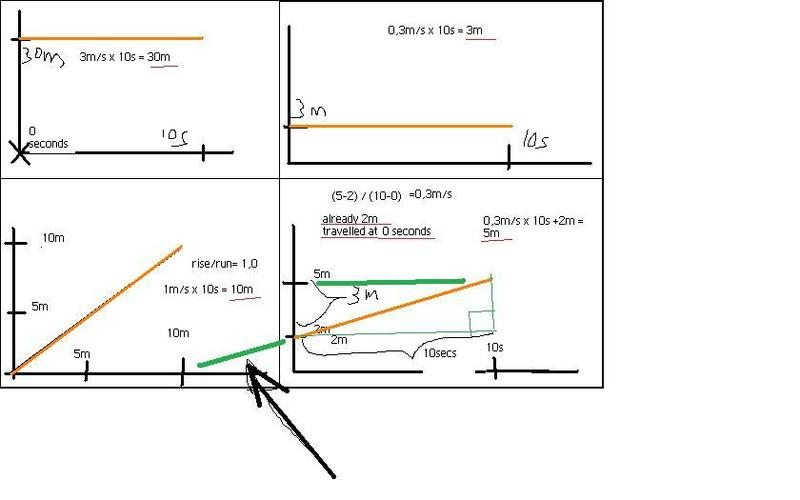# Reading the graph: find distances travelled

## Homework Statement[/B]
there are four moving objects, whose graphs are pictured in orange color.
I forgot to mention that the image, from the book, shows

(y-axis) is denoted by x metres.

The (x axis) is denoted by t seconds.

One could say that these hint towards the idea, that distance travelled was the requested characteristic by the questioner.

Therefore the coordinate system is place, with respect to time (I think this is what the book is telling me)

a) find which object has moved the most during 10 seconds.
b) find which object has moved the least during 10 seconds.

v=s/t velocity

v=d/t speed

## The Attempt at a Solution

objects shall be from upper left-->upper right-->lower left-->lower right
a; b; c; d;

It is difficult to say how one should interpret the graph I think. I think I calculated the velocities wrong in the image.

One could say I suppose that one should use speed equation instead of the velocity equation.

Objects A, and B have been stagnant in their movement because the place is not increasing with time. However A and B distance begin from a number which is >0. 30m distance was already clocked-in at 0 seconds. In case of B, we can see that 3m distance was already clocked in at 0 seconds.

One could argue that within the time period of 0-10s, the already clocked-in amount of distance should be summed up and counted. If you have a foot race with another person for 30m. Let's say that runner R starts the race from 0m marker. However, runner S starts the race from 0+30m marker. Of course, Runner S is at a great advantage. Runner S wins the competition instantly. Then again the physical distance that Runner S runs, is actually only 0m. Seems kind of a silly idea to have a competition like that. But I'm little bit stumped about the question because of that.

A seems to be the graph with the most distance. One can see from the graph that 0-10s is the timeline.
We can see that 30m is the beginning of the distance. We can see that the object is not moving in the cases of A and B. And they are remaining still at the distances of 30m and 3m respectively.

However the graph starts at 30m already clocked-in as distance. One could argue either that the distance is 30m because it was already clocked-in. Or, one could argue that no additional movement happened. Because no additional distance happened.

Andrew Mason
Homework Helper
Your graphs and statement of the problem are confusing. Can you post the actual graphs and actual wording of the questions?

It appears that the y axis represents distance, not speed. If y does not change with time, what does that tell you about its speed?

AM

Your graphs and statement of the problem are confusing. Can you post the actual graphs and actual wording of the questions?

It appears that the y axis represents distance, not speed. If y does not change with time, what does that tell you about its speed?

AM

Is it different in physics when you say: distance travelled, or place? I think I got mixed up with that type of thinking myself, in the beginning. I should have read the instruction more carefully. Originally in the book it says that: The y-axis does denote place ( x metres). The x-axis does denote time (t seconds).

Now that I think about it... I suppose in normal life, distance is defined by essentially the subtraction of two points. So if you have point at 5m and point at 45m, the distance is what is left in between the points really... which would be 40m. Mathematically you could even put the absolute value signs into that one.

if the two points are the same point. Then the distance between them should be zero. I suppose that's true in the absolute value definition on the number line.

Originally in the book it says that: The y-axis does denote place ( x metres). The x-axis does denote time (t seconds).

The questions translated are:

1)which object has moved the most during 10 seconds.
2)which object has moved the least during 10 seconds.

answer 1) I suppose that when the velocity is 0 as it is with uppermost objects. Then the distance is not being travelled at all. Ground is not being treaded forwards. Despite the graph starting from the point at 30m for instance. Therefore the object which moves the most is the lower left corner object moving at the constant speed 1m/s. Distance of that object is 10m travelled within 10s. The lower right corner object does also tread ground within 10s time. The object apparently moves forward as the orange graph indicates. 3m/10s = 0,3m/s. Distance of that object would be 0,3m/s x 10s = 3m distance travelled.

2) objects which move the least during 10 seconds are both the uppermost objects. Their velocities are 0. They do not tread any ground during 10 seconds time which are under review in the graph and the coordinates.